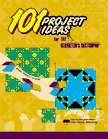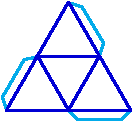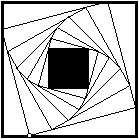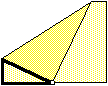Home > General Resources > 101 Project Ideas

# 101 Project Ideas101 Project Ideas for The Geometer's Sketchpad is a booklet from McGraw-Hill Education that's full of exciting project ideas for use in the classroom or at home. The projects are for users with varying degrees of Sketchpad experience and cover a wide range of subject areas (art/animation, triangles, real-world modeling, calculus, transformations and tessellations, trigonometry, fractals, and many more). It's available as a free download (PDF).

## 16 Example Projects

Click links in the project sections below to download example "solution sketches" to sample projects chosen from 101 Project Ideas for The Geometer's Sketchpad. These sketches are designed for both Macintosh and Windows compatibility. Alternately, you can access all these 16 projects in this single Sketchpad document.

###Project 4

A two-dimensional template for folding a three-dimensional solid is called a net. Construct nets for each of the five Platonic Solids, complete with folding tabs. (A net for a tetrahedron is shown at right.) Then print out your nets and build the solids!

###Project 7

The logo for the Interactive Mathematics Program textbook series is shown at right (with a point added). Construct this logo in Sketchpad so that the ratio of a side of a square to the side of the next smallest square remains constant. Animate the point shown bi-directionally along the bottom segment for a really cool effect.

###Project 9

Create two or more figures using segments, arcs, circles, or whatever. The figures can be letters, geometric figures, or even caricaturized faces. Now, using Movement buttons (or, for more control and sophistication, a slider controlling a dilation from one state to the other), set things up so that one figure "morphs" into the other.

### Project 12

Create a sketch that shows the graph of a general cubic function and the graphs of its first and second derivatives. Sliders should control the parameters for the original function, and as they are dragged all three graphs should change accordingly.

### Project 15

Construct a circle and an inscribed angle. Measure the angle and the arc it intercepts. Animate the vertex of the inscribed angle to demonstrate that every inscribed angle that intercepts this arc has the same measure.

###Project 26

If you've read the book Jurassic Park, you're familiar with the Dragon Fractal: it appears at the beginning of each chapter at higher and higher iterations. This fractal is constructed as follows: start with two congruent segments at 90° to each other. Now rotate the entire figure 90° around one of the endpoints. Take the new figure and rotate it 90° around one of its endpoints. Continue this process as many times as you'd like. Try using the Iterate command to quickly generate higher-level versions of the fractal.

### Project 33

Make a sketch that graphs a parabola in standard form y = ax² + bx + c, where a, b, and c are controlled by sliders.

Project 34

The vertex of the parabola y = ax² + bx + c traces another curve when b is allowed to vary (and a and c remain constant). Create a sketch that traces this curve. What is the equation of this function in terms of a, b, and c from the original parabola?

###Project 36

Explore the polar curves r = a + bsin(c·θ) and r = a + bcos(c·θ) by putting Sketchpad into polar mode, setting up sliders for the parameters a, b, and c, and then graphing. (You may want to limit c to integer values using the Calculator's round function. Then again, you may not!) Summarize the effect that each parameter has in the resulting sketch.

### Project 51

Construct a quadrilateral so that the only property it must have is that its diagonals are the same length as each other. What special kinds of quadrilateral could this shape be?

### Project 57

In 1956, the famous philosopher Ludwig Wittgenstein proposed a thought experiment that can be paraphrased as follows:

Imagine that a rod of a given length is attached at one end to a circular track and passes through a fixed pivot point. As the one endpoint moves along the circular track, what kind of curve does the other endpoint describe?

Model this situation in Sketchpad, and then investigate how your results depend on the size of the circular track, the length of the rod, and the location of the pivot point.

###Project 64

When the top-left corner of a piece of paper is folded down to touch the bottom side of the paper, a triangle is formed in the lower-left corner, as shown at left. Model this situation in Sketchpad. (The most challenging thing is for there to be a smooth transition between different states as the point is dragged!) Now use the sketch to determine what fold angle produces the maximum area of the lower-left triangle.

Further challenge: Your sketch probably falls apart when the point is dragged too close to the lower-right corner. Extend your sketch to show (and smoothly transition into) this third possible state. Can you construct a sketch in which the corner point can be dragged all the way around the edge of the piece of paper?

###Project 73

Explore the so-called Lissajou curves, namely the graphs of the parametric equations x(t) = cos(a·t) and y(t) = sin(b·t), where a and b are integers.

### Project 78

Demonstrate two different ways to tile the plane with copies of any arbitrary triangle.

### Project 90

Create a sketch that demonstrates why it is that Side-Side-Angle (SSA) is not a congruence postulate.